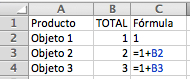# Calculate Accumulated Total in Excel

Learn how to do accumulative additions in Excel with this simple formula for accumulative totals.To calculate an accumulated total is very simple with Excel. If what you need to do is add values to previous ones so they collocate (for example, for inventory), what you need to do is an addition to increase the value of each figure to the previous values.For example, if we imagine that we have a list of elements that we want to add, we place each one into its own row and create a formula that will add the value of every new element (by defect 1) to the value in the cell above it. With each new element that is added, the formula should be dragged to the cell below so that Excel continues to add the value of the cell above onto it. (view example)

In this way we can calculate the accumulative total for each line.

View Excel functions.